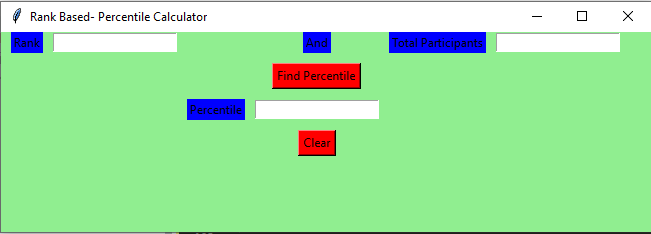Related Articles

# Rank Based Percentile Gui Calculator using Tkinter

• Last Updated : 19 Jan, 2021

Prerequisites:

Python offers multiple options for developing a GUI (Graphical User Interface). Out of all the GUI methods, Tkinter is the most commonly used method. In this article, we will learn how to create a Rank Based – Percentile Gui Calculator application using Tkinter, with a step-by-step guide.
To create a Tkinter:

• Importing the module – Tkinter
• Create the main window (container)
• Add any number of widgets to the main window.
• Apply the event Trigger on the widgets.

The GUI would look like below:Let’s create a GUI based simple Rank Based – Percentile Gui Calculator application :

Below is the implementation :

## Python3

 `# import all functions from the tkinter   ``from` `tkinter ``import` `*` ` ` `# Function to calculate the percentile ``def` `getPercentile() :`` ` `    ``# take a value from the respective entry boxes``    ``# get method returns current text as string``    ``students``=` `int``(total_participantField.get())``     ` `    ``rank ``=` `int``(rankField.get())`` ` `    ``# variable to store the result upto 3``    ``# decimal points ``    ``result ``=` `round``((students ``-` `rank) ``/` `students ``*` `100``,``3``);`` ` `    ``# insert method inserting the  ``    ``# value in the text entry box.``    ``percentileField.insert(``10``, ``str``(result))``   ` `     ` `# Function for clearing the  ``# contents of all text entry boxes``def` `Clear():``     ` `    ``# deleting the content from the entry box``    ``rankField.delete(``0``, END)``     ` `    ``total_participantField.delete(``0``, END)``     ` `    ``percentileField.delete(``0``, END)``     ` ` ` `# Driver Code``if` `__name__ ``=``=` `"__main__"` `:`` ` `    ``# Create a GUI window``    ``gui ``=` `Tk()``   ` `    ``# Set the background colour of GUI window  ``    ``gui.configure(background ``=` `"light green"``)``   ` `    ``# set the name of tkinter GUI window ``    ``gui.title(``"Rank Based- Percentile Calculator"``)``   ` `     ``# Set the configuration of GUI window``    ``gui.geometry(``"650x200"``)`` ` `    ``# Create a Rank: label ``    ``rank ``=` `Label(gui, text ``=` `"Rank"``, bg ``=` `"blue"``)`` ` `    ``# Create a And: label ``    ``andl ``=` `Label(gui, text ``=` `"And"``, bg ``=` `"blue"``)``   ` `    ``# Create a Total Participants : label``    ``total_participant ``=` `Label(gui,``                              ``text ``=` `"Total Participants"``,``                              ``bg ``=` `"blue"``)`` ` `    ``# Create a Find Percentile Button and``    ``# attached to getPercentile function``    ``find ``=` `Button(gui, text ``=` `"Find Percentile"``,``                  ``fg ``=` `"Black"``, bg ``=` `"Red"``,``                  ``command ``=` `getPercentile)``     ` `    ``# Create a Percentile : label ``    ``percentile ``=` `Label(gui, text ``=` `"Percentile"``, bg ``=` `"blue"``)`` ` `    ``# Create a Clear Button and attached``    ``# to Clear function``    ``clear ``=` `Button(gui, text ``=` `"Clear"``,``                   ``fg ``=` `"Black"``, bg ``=` `"Red"``,``                   ``command ``=` `Clear)`` ` `    ``# grid method is used for placing  ``    ``# the widgets at respective positions  ``    ``# in table like structure .`` ` `    ``# padx attributed provide x-axis margin ``    ``# from the root window to the widget.``    ``rank.grid(row ``=` `1``, column ``=` `1``,padx ``=` `10``)`` ` `    ``andl.grid(row ``=` `1``, column ``=` `4``)``                  ` `    ``total_participant.grid(row ``=` `1``, column ``=` `6``, padx ``=` `10``)`` ` `    ``# pady attributed provide y-axis``    ``# margin from the widget.   ``    ``find.grid(row ``=` `3``, column ``=` `4``,pady ``=` `10``)``     ` `    ``percentile.grid(row ``=` `4``, column ``=` `3``,padx ``=` `10``)``     ` `    ``clear.grid(row ``=` `5``, column ``=` `4``,pady ``=` `10``)`` ` `    ``# Create a text entry box for filling or``    ``# typing the information.  ``    ``rankField ``=` `Entry(gui)``     ` `    ``total_participantField ``=` `Entry(gui)``     ` `    ``percentileField ``=` `Entry(gui)`` ` `    ``# grid method is used for placing  ``    ``# the widgets at respective positions  ``    ``# in table like structure .``    ``rankField.grid(row ``=` `1``, column ``=` `2``)``     ` `    ``total_participantField.grid(row ``=` `1``, column ``=` `7``)``     ` `    ``percentileField.grid(row ``=` `4``, column ``=` `4``)``     ` `    ``# Start the GUI``    ``gui.mainloop()`

Output :

Attention geek! Strengthen your foundations with the Python Programming Foundation Course and learn the basics.

To begin with, your interview preparations Enhance your Data Structures concepts with the Python DS Course. And to begin with your Machine Learning Journey, join the Machine Learning – Basic Level Course

My Personal Notes arrow_drop_up